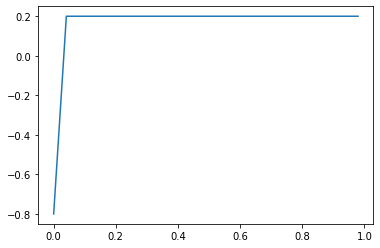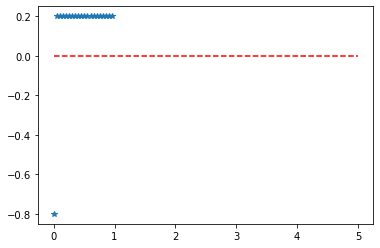# Python – Uniform Discrete Distribution in Statistics

• Last Updated : 10 Jan, 2020

scipy.stats.randint() is a uniform discrete random variable. It is inherited from the of generic methods as an instance of the rv_discrete class. It completes the methods with details specific for this particular distribution.

Parameters :

Attention geek! Strengthen your foundations with the Python Programming Foundation Course and learn the basics.

To begin with, your interview preparations Enhance your Data Structures concepts with the Python DS Course. And to begin with your Machine Learning Journey, join the Machine Learning - Basic Level Course

x : quantiles
loc : [optional]location parameter. Default = 0
scale : [optional]scale parameter. Default = 1
moments : [optional] composed of letters [‘mvsk’]; ‘m’ = mean, ‘v’ = variance, ‘s’ = Fisher’s skew and ‘k’ = Fisher’s kurtosis. (default = ‘mv’).

Results : uniform discrete random variable

Code #1 : Creating uniform discrete random variable

 `# importing library`` ` `from` `scipy.stats ``import` `randint ``   ` `numargs ``=` `randint .numargs ``a, b ``=` `0.2``, ``0.8``rv ``=` `randint (a, b) ``   ` `print` `(``"RV : \n"``, rv)  `

Output :

```RV :
scipy.stats._distn_infrastructure.rv_frozen object at 0x0000016A4D865848
```

Code #2 : uniform discrete variates and probability distribution

 `import` `numpy as np ``quantile ``=` `np.arange (``0.01``, ``1``, ``0.1``) `` ` `# Random Variates ``R ``=` `randint .rvs(a, b, size ``=` `10``) ``print` `(``"Random Variates : \n"``, R) `` ` `# PDF ``x ``=` `np.linspace(randint.ppf(``0.01``, a, b),``                ``randint.ppf(``0.99``, a, b), ``10``)``R ``=` `randint.ppf(x, ``1``, ``3``)``print` `(``"\nProbability Distribution : \n"``, R) `

Output :

```Random Variates :
[ 3  0  0 15  0  1  4  2  0  6]

Probability Distribution :
[1. 1. 1. 1. 1. 1. 1. 1. 1. 1.]

```

Code #3 : Graphical Representation.

 `import` `numpy as np ``import` `matplotlib.pyplot as plt ``    ` `distribution ``=` `np.linspace(``0``, np.minimum(rv.dist.b, ``2``)) ``print``(``"Distribution : \n"``, distribution) ``    ` `plot ``=` `plt.plot(distribution, rv.ppf(distribution)) `

Output :

```Distribution :
[0.         0.04081633 0.08163265 0.12244898 0.16326531 0.20408163
0.24489796 0.28571429 0.32653061 0.36734694 0.40816327 0.44897959
0.48979592 0.53061224 0.57142857 0.6122449  0.65306122 0.69387755
0.73469388 0.7755102  0.81632653 0.85714286 0.89795918 0.93877551
0.97959184 1.02040816 1.06122449 1.10204082 1.14285714 1.18367347
1.2244898  1.26530612 1.30612245 1.34693878 1.3877551  1.42857143
1.46938776 1.51020408 1.55102041 1.59183673 1.63265306 1.67346939
1.71428571 1.75510204 1.79591837 1.83673469 1.87755102 1.91836735
1.95918367 2.        ]
```Code #4 : Varying Positional Arguments

 `import` `matplotlib.pyplot as plt ``import` `numpy as np `` ` `x ``=` `np.linspace(``0``, ``5``, ``100``) ``    ` `# Varying positional arguments ``y1 ``=` `randint.ppf(x, a, b) ``y2 ``=` `randint.pmf(x, a, b) ``plt.plot(x, y1, ``"*"``, x, y2, ``"r--"``) `

Output :My Personal Notes arrow_drop_up